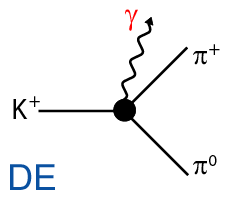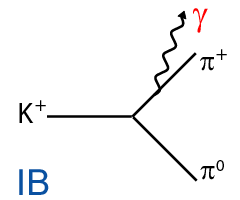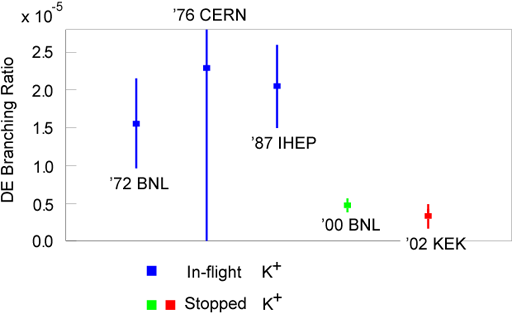## Measurement of direct photon emission in K+ → π+π0γ decay using stopped positive kaons

### Motivation

The chiral anomaly, which is a basic feature of quantum field theory, has been the subject of extensive theoretical investigation. Recently, this chiral anomaly can be treated in the frame work of chiral perturbation theory (ChPT) .
Since the K+ → π+π0 decay is hindered as it violates the Δ I=1/2 rule, the internal bremsstrahlung (IB) to the radiative K+ → π+π0γ (Kπ2γ) decay is also suppressed. This feature, in turn, enhances the direct emission (DE) which is sensitive to the chiral anomaly. Four experiments [ 3, 4, 5, 6] have reported on the DE branching ratio in the region of 55 < Tπ+ < 90 MeV, where Tπ+ is the π+ kinetic energy. These experiments restrict the π+ energy region to remove backgrounds from K+ → π+π0π0 Therefore, we measured the Kπ2γ events by extending the Tπ+ region below the Kπ3 end-point energy Tπ+max = 55 MeV.### Experiment

The experiment was performed at the KEK 12 GeV proton synchrotron in 2001 and we ran the experiment for about 100 shifts (∼ 1 month). The Kπ2γ data were collected with the experimental apparatus based on the E246 experiment searching for T-violation in K+ → π0μ+ν decay. A Pb-plastic sandwich detector with 2.6 radiation length was newly set at the outer radius of the magnet pole to monitor photons escape from the photon detector holes. The kaons were slowed down by a degrader and stopped in the target. The Kπ2γ were identified by analyzing the π+ momentum with the spectrometer and detecting three photons in the CsI(Tl) calorimeter.
The most important point to reconstruct Kπ2γ was to determine which photons were a pair from a π0, because there are three combinations to form the π0. The pair which satisfies the Kπ2γ kinematics was adopted as the correct π0 pair. However, because of the finite kinematical resolution, the correct pairing probability was 85% for IB and 79% for DE. Since Kπ3 and Kπ2 decays do not satisfy the Kπ2γ kinematics, these backgrounds can be rejected by this analysis.### Results

 The number of good Kπ2γ events is 4434. The experimental Kπ2γ spectra are shown in Figure. In order to obtain the detector acceptance and response functions, the Monte Carlo simulation was performed using a GEANT-based Monte Carlo code. The background fraction of the Kπ3 decay was estimated to be 1.2% from the simulation. The DE branching ratio was determined using the three observables, cosθπ+γ, cosθπ0γ, and Eγ which have characteristic spectra corresponding to the decay processes. The experimental distribution ρ(cosθπ+γ, cosθπ0γ, Eγ) was fitted to the simulation spectrum of IB[1+α(DE/IB)] with α being free parameters. The fitted spectra and the sole DE components are also shown in Figure. We determined the DE branching ratio to be  Br(DE, total) = [6.1 ± 2.5(stat.) ± 1.9(syst.)]×10-6 . In order to compare this result with the previous ones, the partial branching ratio in the region of 55 < Tπ+ < 90 MeV was also derived as  Br(DE, partial) = [3.2 ± 1.3(stat.) ± 1.0(syst.)] ×10-6 which is consistent with the previous stopped experiment. Figure: Kπ2γ spectra: (a) π+momentum, (b) opening angle between π+ and γ3, (c) opening angle between π0 and γ3, (d) γ3 energy.### Reference

 S.L. Adler, Phys. Rev. 177 (1969) 2426; J.S. Bell and R. Jackiw, Nuovo Cimento 60A (1969) 47; W.A. Bardeen, Phys. Rev. 184 (1969) 1848.
 A. Buras, Theoretical review of K-physics, hep-ph/9609324; A. Pich, Rare kaon decays, hep-ph/9610243.
 R.J. Abrams et al., Phys. Rev. Lett. 29 (1972) 1119.
 K.M. Smith et al., Nucl. Phys. B109 (1976) 173.
 V.N. Bolotov et al., Sov. J. Nucl. Phys. 45 (1987) 1023.
 S. Adler et al., Phys. Rev. Lett. 85 (2000) 4856.
 M. Abe et al., Phys. Rev. Lett. 83 (1999) 4253.
 G. D'Ambrosio, M. Miragliuolo, and P. Santorelli, in DaΦne PhysicsHandbook, edited by L. Maiani, G. Pancheri, and N. Paver (Laboratori Nazionali di Frascati, Frascati, 1992).
 M.A. Aliev et al., Phys. Lett. B554 (2003) 7.